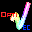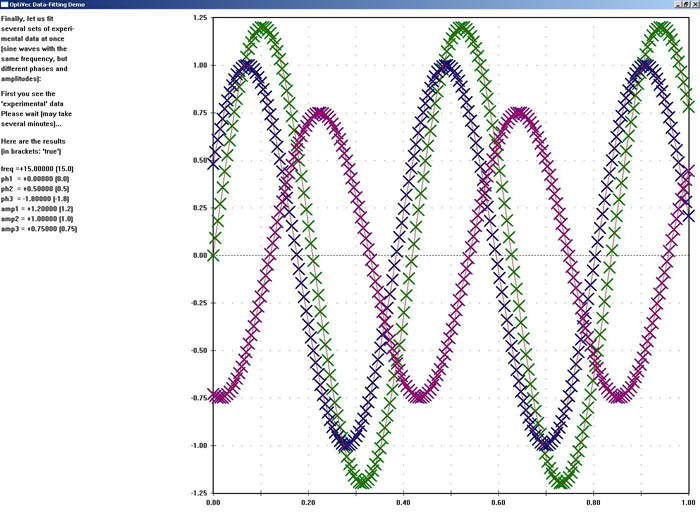# OptiVec for Visual C++

8.0.1
OptiVec: Fast vector and matrix library for MS Visual C++Fast vector and matrix library for Visual C++
OptiVec contains more than 3500 hand-optimized, machine-code-written functions for all floating-point and integer data types from the following fields: 1. Vectorized form of arithmetic operators and math functions. 2. Matrix operations, e.g.: multiplication, inversion, LU decomposition, singular value decomposition, eigenvalues. 3. Fast Fourier Transform techniques for efficient convolutions, correlation analyses, spectral filtering, etc., both one- and two-dimensional. 4. Curve fitting for a wide range of model functions from simple linear regression to non-linear models with multiple data sets. 5. Statistics. 6. Analysis (derivatives, integrals, extrema, interpolation). 7. Graphical representation of data in Cartesian coordinates. 8. Complex number math, both in cartesian and polar format. The vectorized implementation in Assembler makes OptiVec functions, on the average, 2-3 times faster than compiled source code of the same functionality. In many instances, the numerical accuracy is improved as well. Optionally, the object-oriented interface for C++, 'VecObj', offers simplified function calls and increased memory safety. This version is for Microsoft Visual C++ (all version of Visual Studio from the current one down to VS2005).
Technical details
Title:
OptiVec for Visual C++ 8.0.1 for Windows
Requirements:
Microsoft Visual Studio (at least Visual C++ 2005)
OS Support:
Win2000, WinXP, Win7 x32, Win7 x64, Windows 8, Windows 10, WinServer, WinOther, WinVista, WinVista x64
Language:
German, English